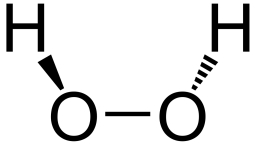# Hydrogen 18823

I have 1 liter of 9% hydrogen peroxide, making it a 3% solution.
How much water do I need to add?

V =  2 l

### Step-by-step explanation:

0.09 · 1 = 0.03·(1+V)

0.03V = 0.06

V = 0.06/0.03 = 2

V = 2

Our simple equation calculator calculates it.Did you find an error or inaccuracy? Feel free to write us. Thank you!

Tips for related online calculators